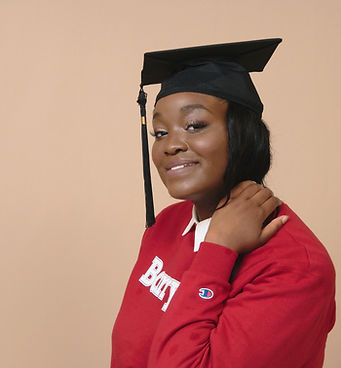top of page# Everest Turner

BS Nutritional Sciences

MS Biomedical Science

Everest Turner is a South Florida native and Southern California implant. After earning her Bachelor's degree in Nutritional and Natural Sciences and Master's degree in Biomedical Science she has committed herself fully to educating the future and building up the next generation of scientific world changers. Today, Everest can be found laying the foundation of knowledge through teaching both K-12 and post-secondary education as well as tutoring all while applying to the Medical Doctorate Program to become a family medicine physician to assist both children and adults with becoming healthier and more wholesome.

## Math Games & Projects 2

Games, Manipulatives and more!

Ages 7-9 and others that are operating at this math level

Prerequisites: Students should be able to do multi-digit addition and subtraction, able to add through 1000.  Students should have been introduced to multiplication.  Know their shapes, and understand perimeter.

Homework:  Optional.  This is designed to supplement your at-home math program.

Math is a natural part of our thinking.  From a young age, children find patterns and think about things mathematically.  In this class we will harness this natural curiosity to learn about math without creating anxiety and/or boredom that many learn in the traditional math class.  Students will learn how to use math language to discuss math problems and be problem solvers.  We will learn from each other’s methods.  This will be an active class, with hands-on investigations, games and longer term projects.

Serious learning will be done in our class – but it will be so much fun they will never know how much they are learning!  Games are the perfect way to adapt learning for different levels while encouraging critical thinking skills and appropriate social skills.

While we will be varying this based on the needs of the class, the main curriculum we will cover is:

Fall:

• Place Value up to billions

• Expanded Form (3x1000+4x100+2x10+4=3,424)

• Negative numbers on number line (add and subtract if capable)

• Multiply and divide by 10, 100, 1000

• Multiplication/Division

• What factors make up numbers (Factors of 20 are 2, 2, 5)

• Multiplying single digits fluently

• Greatest Common Factors (GCF)

• Least Common Multiple (LCM)

• Squares/Square roots (and other exponents if capable)

Winter:

• Multi-digit Multiplication/Division

• Understand that multiplication and division are inverse operations

• Multiplying 2-3 digit factors

• Dividing 3-4 digit quotients

• Geometry

• Area, Volume, Surface Area

• Understand pi and how to use for circular shapes

• Angles

• Graph on coordinated plane

Spring

• Decimals

• To thousandths

• Write in expanded form

• Round

• Fractions

• Add, subtract, multiply, and divide

• Write fractions as decimals

• Simplify fractions

Contact Everest Here:  tutorwitheverest@gmail.com

bottom of page